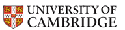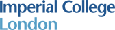# Master Maths

## You can learn how to solve hardest problems and how to write rigorous proofs

### Successful students at top UK universities

• 69 students entered top universities after the courses, read success stories
 Universities Cambridge Oxford Warwick Imperial all students all years 27 13 16 13 69
• 100% students who completed the STEP courses in 2016 were accepted at Cambridge.
• The table contains only confirmed destinations, not all students have replied (one success case from 2012 was confirmed by a school teacher only in 2013).
• Other destinations not counted in the table above include University College London (UCL), King's College London, Universities of Bath, Bristol, Durham, Edinburgh, Sheffield, Southampton, St Andrews, London School of Economics, Columbia University (USA), Waterloo University (Canada).
• Following former students' request, you can ask us about degree level tuition.
• The offered training courses are completely independent of any universities.

### Top UK universities and entrance exams

Top UK universities usually accept students with good STEP grades, please click on the university logos to access maths admissions webpages:• STEP II and III are the Sixth Term Exam Papers in June, see the STEP webpage. The possible STEP grades are S (excellent), 1 (very good), 2 (good), 3 (satisfactory), U (poor).
• Cambridge may ask for STEP II and III, a usual offer is a grade 1 in both papers.
• Past students have gained 18 highest grades S in STEP exams.  years/exams STEP I STEP II STEP III all grades S 2016 1 2 3 6 all years 4 5 9 18

### What you can expect from web tutorials

Each web tutorial covers a long multi-step problem split into 4 parts

• in every part: you may attempt several times an interactive quiz that includes randomised parameters, immediate feedback, progressive hints
• after taking the quiz (even if scored 0), you may review detailed answers
• difficulty of quizzes is increasing in each tutorial, you need all previous steps
• if anything is unclear, you may submit your anonymous feedback at the end
• many tutorial problems can be solved in numerous different correct ways, so you may outline your own method: contact us or send anonymous feedback
• your feedback is seriously considered and the tutorials are regularly updated.

### How to access and work through tutorials

• guests have the read-only access, interactive quizzes work only for enrolled users
• self-register click on Create a New Account, login, navigate to our free tutorial Beyond Pythagoras' theorem and click on enrol me in this tutorial in the left menu
• prepare some draft paper and a pencil for your computations during a tutorial
• if a quiz seems hard, you may finish it and review answers in the next activity
• make frequent breaks and study regularly: learning in small portions is effective

### The list of 49 available web tutorials (log in as a guest for a quick view):

• Free tutorial: Beyond Pythagoras' theorem and applications
• 4 tutorials on Oxbridge interviews (log in as a guest): prices
• Combinatorial choices of rooks, words and signs
• Rates of increase, cubes of roots and binary numbers
• Properties of primes, factorials, recurrent sequences
• Counting roots of complicated graphs of functions
• 8 tutorials on STEP II exam 2013 (log in as a guest): prices
• Areas under graphs help prove inequalities
• Converging non-monotone recurrent sequences
• Signs of roots and graphs of cubic polynomials
• Graphs of rational functions with a double symmetry
• Diaphontine equations with infinitely many solutions
• Using graphs to compare powers with arbitrary bases
• Sketching loci of middle points of variable chords
• Recursive integration of functions with parameters
• 16 tutorials on STEP I exam 2011-2012 (log in as a guest): prices
• Minimum distances between points on the plane
• Periodic recurrent sequences with a 3-term relation
• The graphs and real roots of quartic parabolas
• Symmetric tangents and normals to a hyperbola
• Homogeneous first order differential equations
• Integrating trigonometric functions with a logarithm
• Inequalities between areas under graphs of functions
• Angles of elevation in a pyramid over a right-angled triangle
• Tangents and normals to transformed hyperbolas
• Two intersecting tangents to a horizontal parabola
• Trigonometric identities with products and sums
• Graphs and extrema of trigonometric functions
• Basic integration by substitution and by parts
• Evaluations of infinite binomial expansions
• Diaphontine equations with finitely many solutions
• Differential equations of flows and leaks in a bath
• 20 tutorials on MAT 2011-12 (log in as a guest): prices
• Algebraic expressions: squares, equations, estimates
• Tangent lines to circles and graphs of polynomials
• Computing areas of triangular regions in a disk
• Integration and areas under graphs of functions
• Repeated compositions of two linear functions
• Turning points and the area under a cubic parabola
• How a circle and a parabola can touch each other
• A cyborg executing a computer-generated code
• Logical analysis of exam results in a large class
• Best strategies to win a number game modulo 3
• Counting combinatorial choices: jumps and wins
• Inequalities and recurrence relations for sequences
• Recurrence relations between multiple sequences
• Equations: polynomial, trigonometric, exponential
• Plane geometry: rectangles, circles and angles
• Sketching graphs of functions and applications
• Tangent lines and areas bounded by cubic parabolas
• Global extrema of a 2-variable function over a region
• Logical analysis of multiple claims for crime detection
• Linear pattern recognition in competitive games